# Equation of a Straight Line

Equation of a Straight Line
Go back to  'Coordinate Geometry'

Do you know that the equation of a line is really great to describe all sort of situations around you?

For example, Harry has just turned 18 and his parents gifted him a car on his birthday.The car has 27000 miles in it and every year he will travel 12,000 miles to his college.

We can represent it in the form of an equation of line as $$y=27000x+12000$$.

In this mini-lesson, we will explore the world of the equation of a straight line. We will try to understand the general equation of a line, straight line formula, the way of finding the equation of a straight line and discover other interesting aspects of it. You can check out the interactive simulations to know more about the lesson and try your hand at solving a few interesting practice questions at the end of the page.

We don't want you to worry, as we, at Cuemath, understand how this topic can be confusing for you.

We are here to help you understand this concept in a clear way.

## What Is the Equation of a Straight Line?

### Straight Line: Definition

A straight line is a figure formed when two points $$A (x_1 , y_1)$$ and $$B (x_2 , y_2)$$ are connected with minimum distance between them, and both the ends extended to infinity.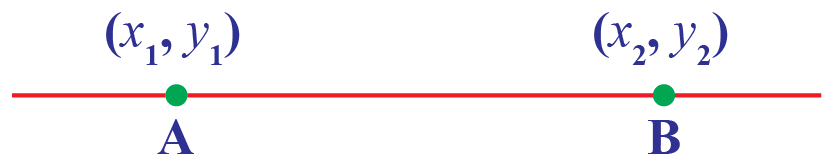### Straight Line Formula

The standard form of a linear equation with variables $$x$$ and $$y$$ is:

 $ax + by = c$

where $$a, b, c$$ are constants and $$x, y$$ are variables.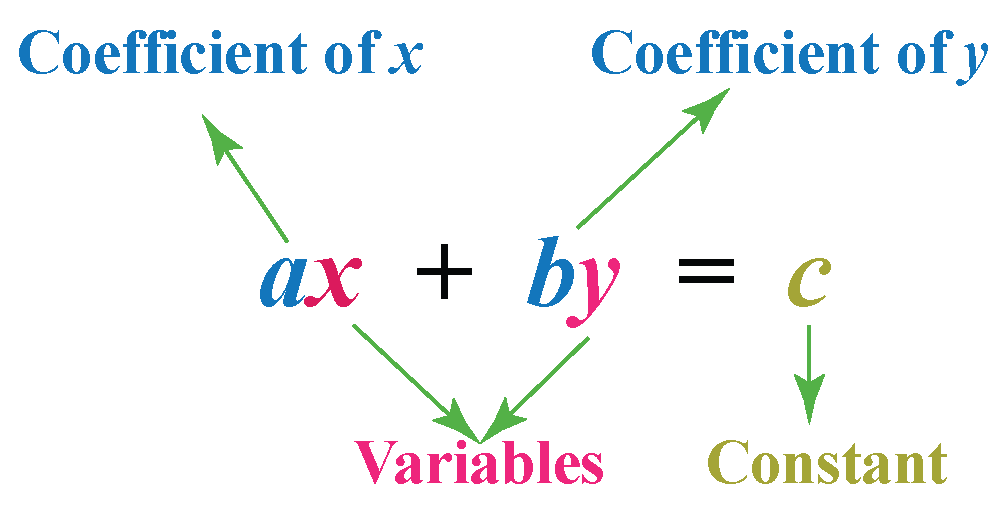## The Slope of a Straight Line

When you draw a line in XY-plane, have you observed that the line forms two angles with $$x$$ axis which are supplementary?

Suppose a line $$l$$ makes an angle of $$\theta$$ with a positive direction of $$x$$ axis.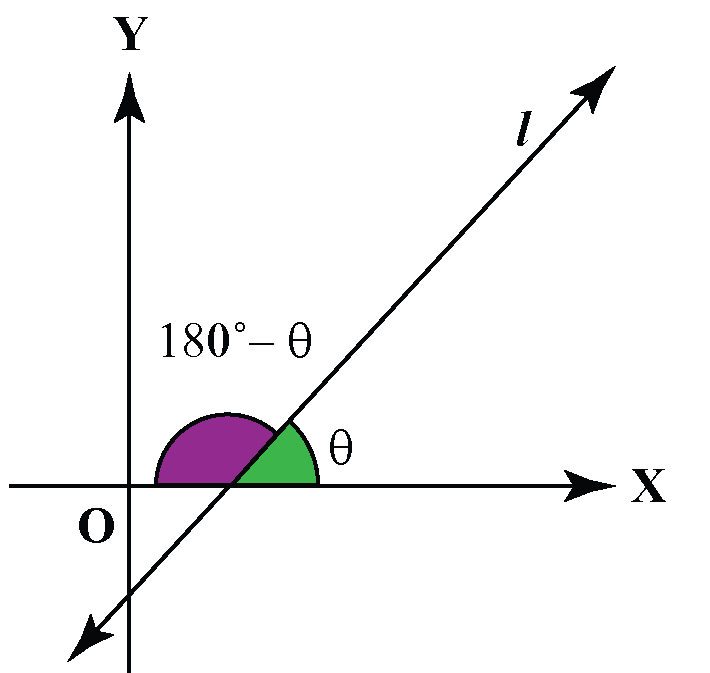The angle $$\theta$$ is called the inclination of the line and $$\tan{\theta}$$ is called the slope of the line.

Note that $$x$$-axis has the slope 0. In fact, all lines parallel to $$x$$-axis have the slope 0.

Also, the slope of all the vertical lines including $$y$$-axis is not defined.

### Slope Formula

Suppose we have a line joining two points $$(x_1,y_1)$$ and $$(x_2,y_2)$$ having inclination of $$\theta$$.

Then, the slope $$m$$ of the line is given by:

 $$m=\dfrac{y_2-y_1}{x_2-x_1}$$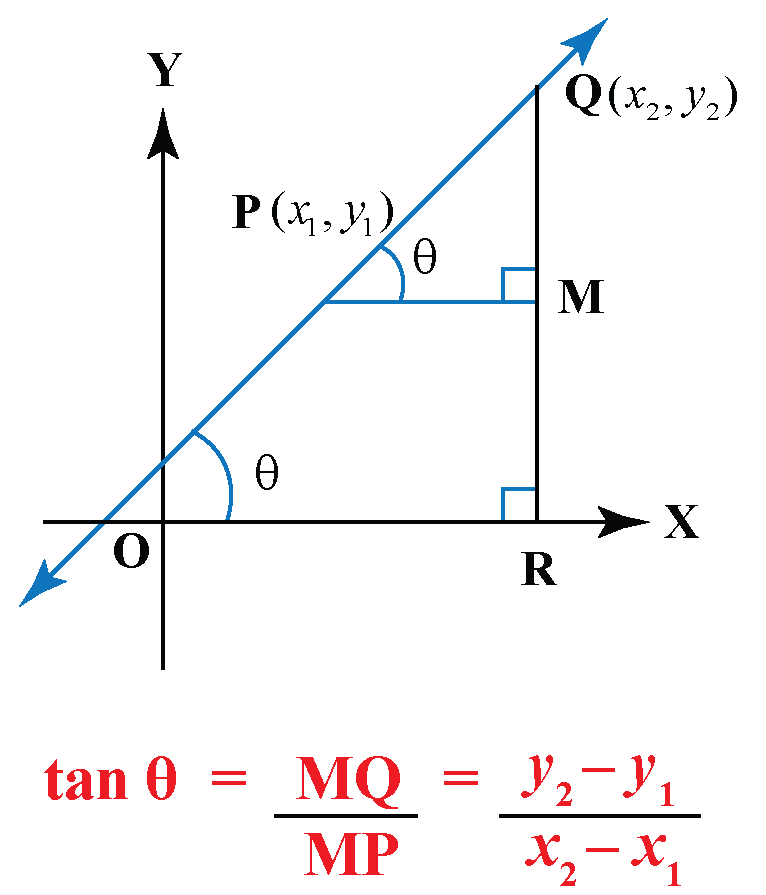## How To Find the Equation of a Straight Line?

### Using General Form

The general form of a straight line is given by $$ax+by=c$$.Let's transform the equation $$y=2x-1$$ in the general form.

Add 1 to both sides of the equation.

So, we obtain the general form as $$2x-y=1$$.

Can you transform $$y=-\dfrac{x}{2}+7$$ in the general form?

### Using Standard Form

What if you are given just two points $$(x_1, y_1$$) and $$(x_2, y_2)$$ and you have to find the equation of a straight line joining these two points?

Let us take a point $$(x,y)$$ lying between $$(x_1, y_1$$) and $$(x_2, y_2)$$ on the line.

Notice that these three points are collinear.

So, we can write

\begin{align}\dfrac{y-y_1}{x-x_1}&=\dfrac{y_2-y_1}{x_2-x_1}\\y-y_1&=\left(\dfrac{y_2-y_1}{x_2-x_1}\right)(x_2-x_1)\end{align}

The equation of the standard form is:

 $$y-y_1=\left(\dfrac{y_2-y_1}{x_2-x_1}\right)(x_2-x_1)$$

### Using Point-slope Form

The equation of a line whose slope is $$m$$ and which passes through a point $$(x_1, y_1$$) is found using the point-slope form.

The equation of the point-slope form is:

 $${y-y_1=m(x-x_1)}$$

where $$(x, y)$$ is a random point on the line.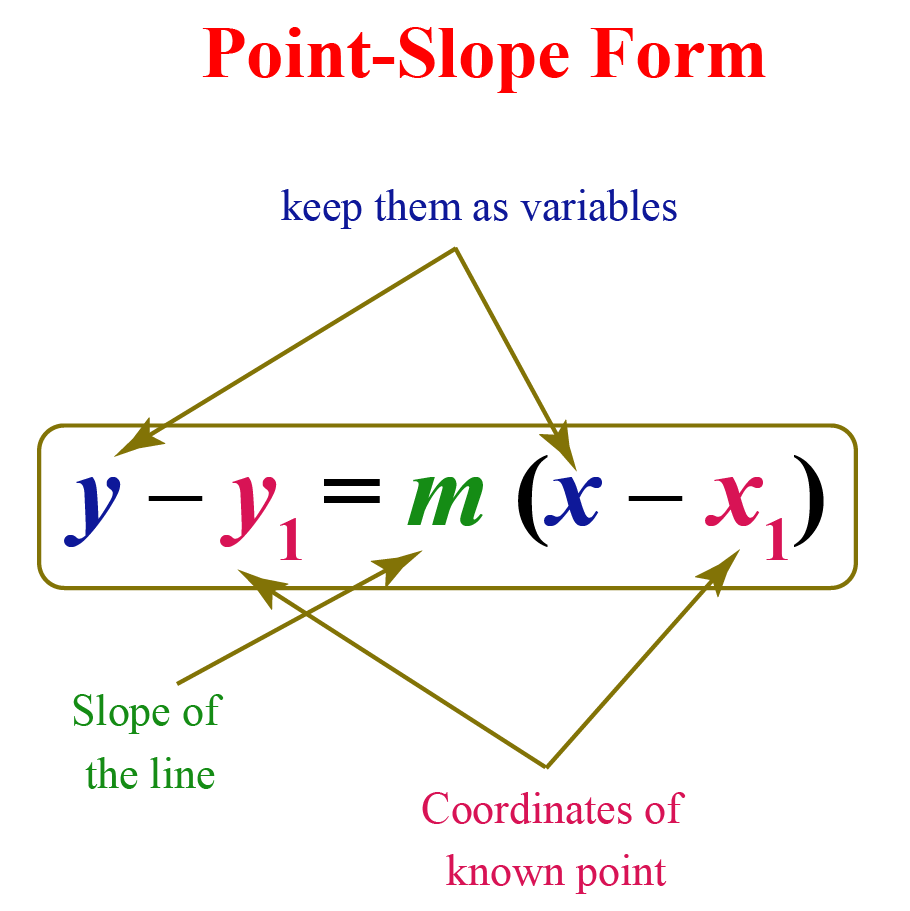Let us see how to find the point-slope form.

We will derive this formula using the equation for the slope of a line.

Let us consider a line whose slope is $$m$$.

Let us assume that $$(x_1, y_1$$) is a known point on the line.

Let $$(x,y)$$ be any other random point on the line whose coordinates are not known.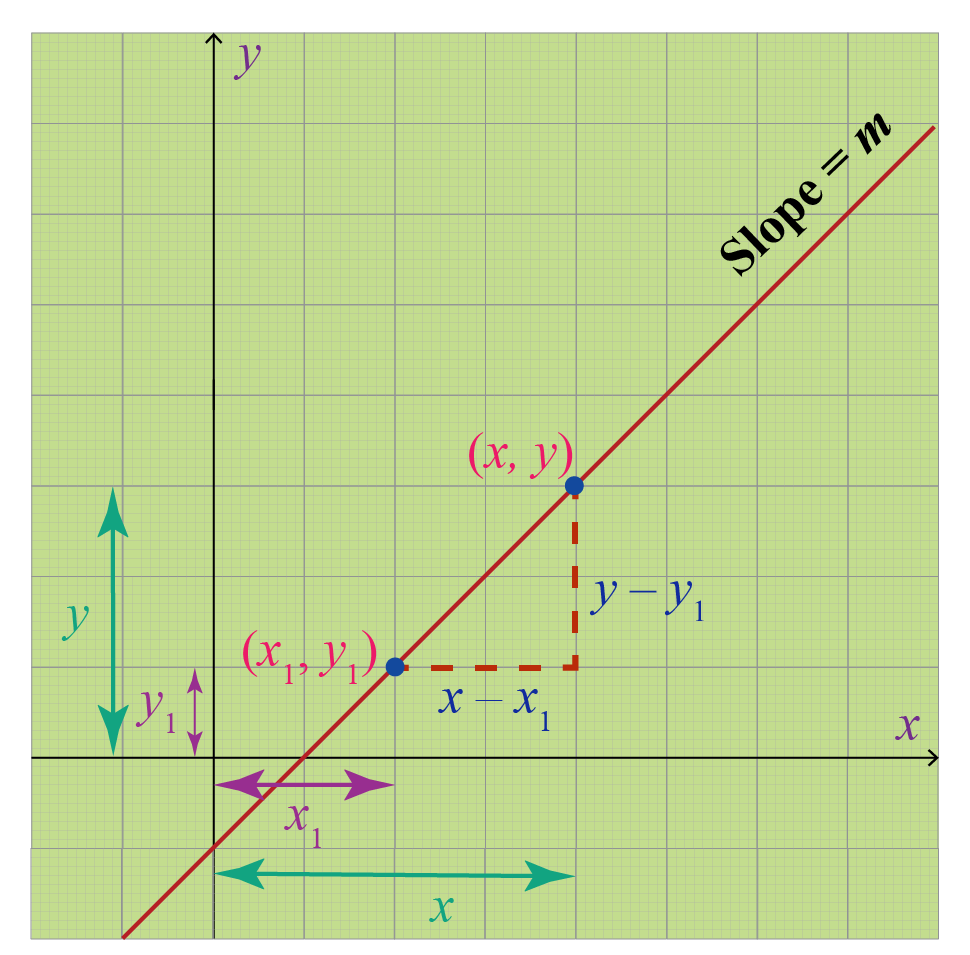We know that the equation for the slope of a line is:

\begin{aligned}\text{Slope }&= \dfrac{ \text{Difference in y-coordinates}}{ \text{Difference in x-coordinates}}\\[0.2cm]m&= \dfrac{y-y_1}{x-x_1} \end{aligned}

Multiplying both sides by $$(x-x_1)$$,

$m(x-x_1) = y-y_1$

This can be written as,

$y-y_1=m(x-x_1)$

Hence the point-slope formula is proved.

### Using Slope-intercept Form

Now, suppose a line is known to you with its slope and an intercept on one of the axes.

Say, a line intersects the $$y$$-axis at the point $$(0,c)$$.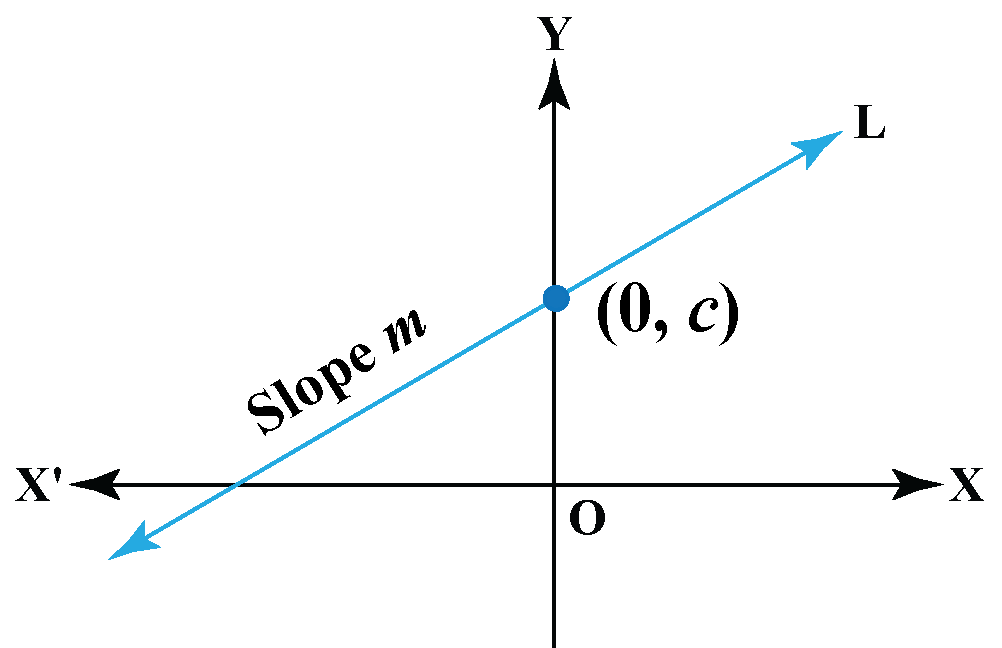Using the point-slope form we have $$y-c=m(x-0)$$ or $$y=mx+c$$.

 $y=mx+c$

where $$c$$ is the $$y$$ intercept.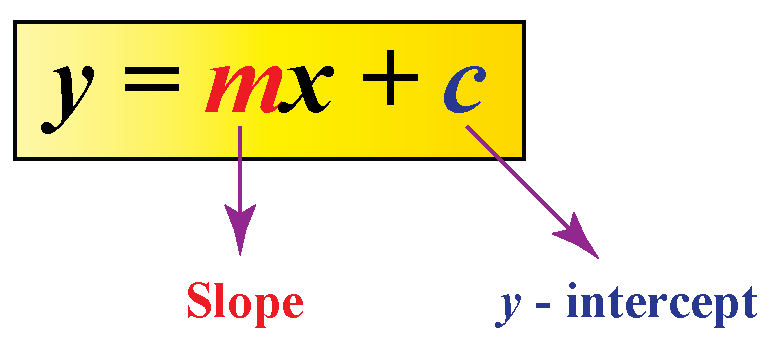Similarly, if $$d$$ is the $$x$$ intercept, then slope-intercept form is:

 $y=m(x-d)$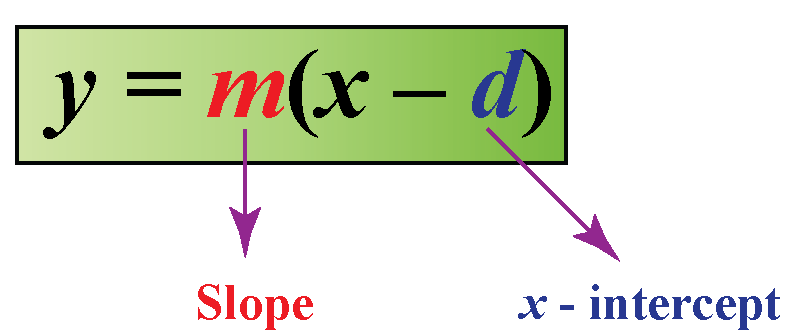## Equations of Straight Line on Graph

The graph of a linear equation in one variable $$x$$ forms a vertical line parallel to $$y$$-axis and vice-versa.

The graph of a linear equation in two variables $$x$$ and $$y$$ forms a straight line.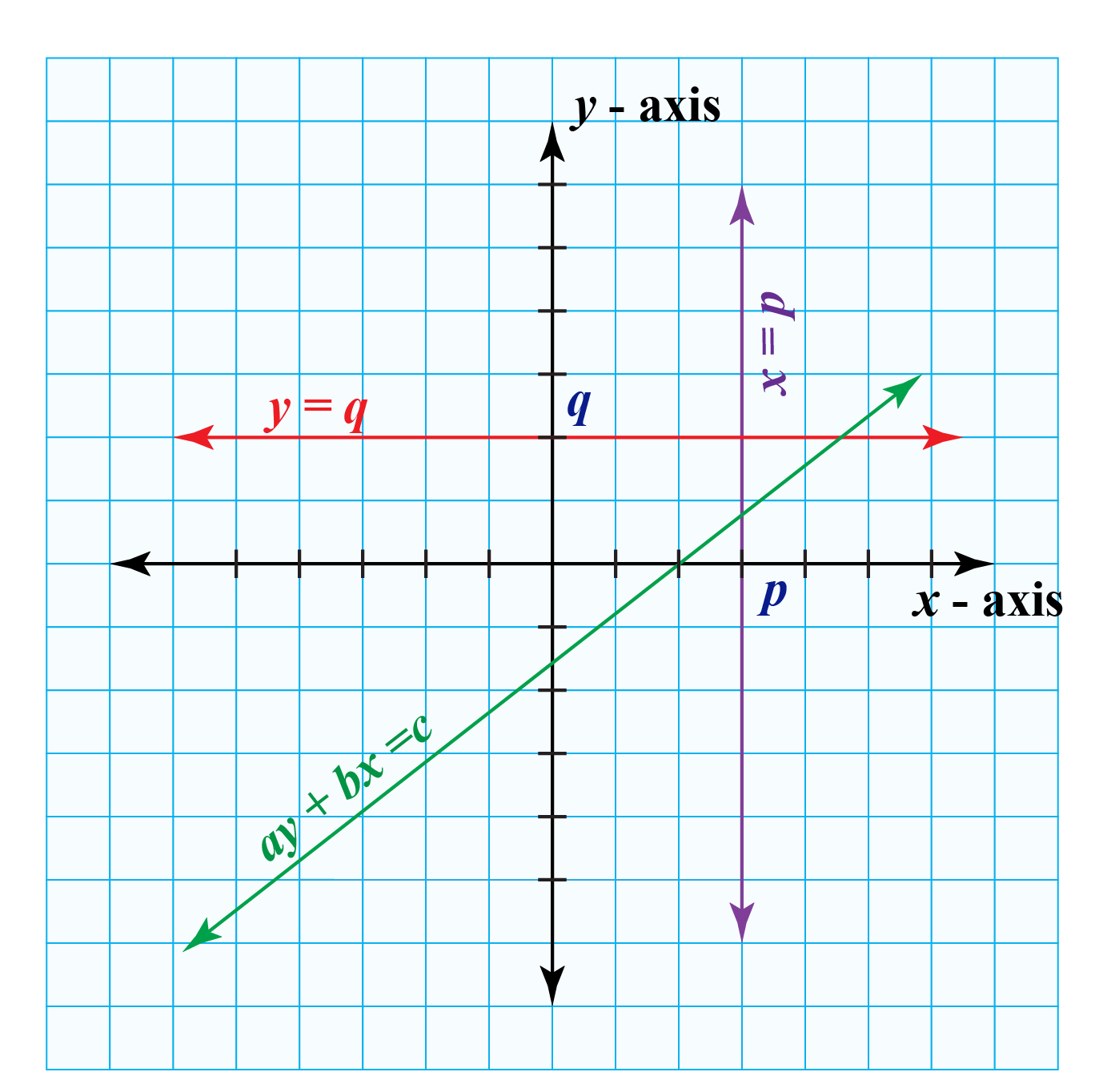Explore the graphs of equations of a straight line using the simulation below.

## Solved Examples

 Example 1

Carlos will begin attending classes for the sixth grade from next week.

He suddenly realized that he doesn't have either a notebook or a pen.

He goes to a shop to purchase them and realizes that the cost of a notebook is twice more than $5 for a pen.Represent this above information using the equation of a straight line. Solution Let's assume the following. The cost of a pen =$$$x$$

The cost of a notebook = \$$$y$$

According to the given information,

 $$\therefore$$ The equation of straight line is $$y=2x +5$$.
 Example 2

Look at the population and year graph.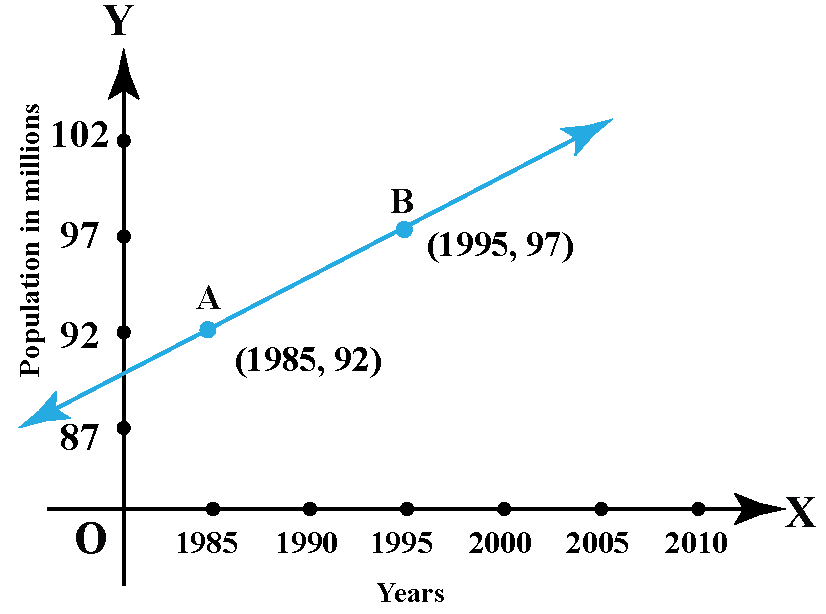Can you determine the population in the year 2010 using the slope of the line AB?

Solution

The line AB passes through $$A(1985, 92)$$ and $$B(1995, 97)$$.

Let $$x_1=1985, y_1=92, x_2=1995$$ and $$y_2=97$$.

Using the slope equation we can calculate the slope of the line AB.

\begin{align}\text{Slope of AB}&=\dfrac{y_2-y_1}{x_2-x_1}\\&=\dfrac{1995-1985}{97-92}\\&=\dfrac{1}{2}\end{align}

Let the population in the year 2010 be $$z$$.

Then, the line AB must pass through $$C(2010,z)$$.

\begin{align}\text{Slope of AB}&=\text{Slope of BC}\\\dfrac{1}{2}&=\dfrac{z-97}{2010-1995}\\\dfrac{15}{2}&=z-97\\z&=104.5 \end{align}

 $$\therefore$$ Population in year 2010 is 104.5 millions.
 Example 3

Jolly's teacher asked her to find the equation of a line passing through two points as shown on the blackboard.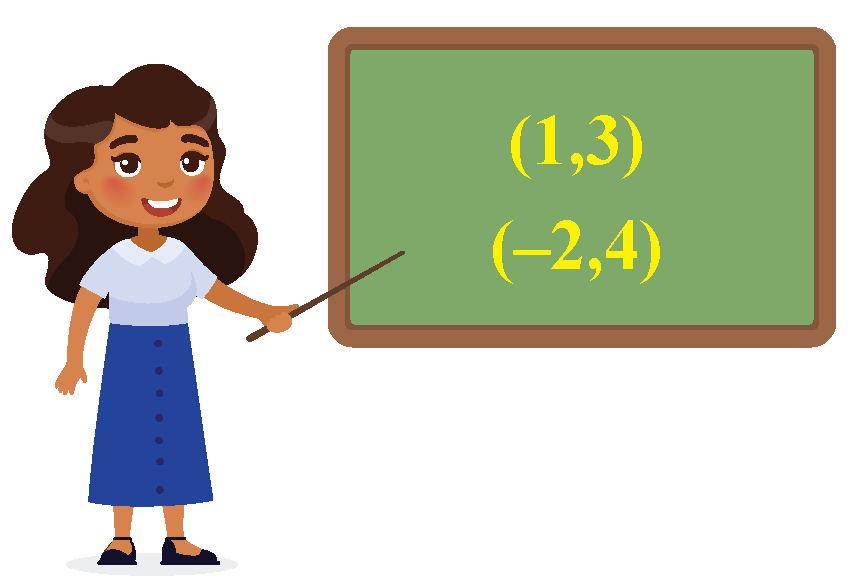Can you help her?

Solution

The given two points are:

\begin{aligned} (x_1, y_1)&=(1,3)\\[0.2cm] (x_2,y_2)&=(-2, 4) \end{aligned}

To use the point-slope form, we first need to find the slope of the line.

The slope of the line is found using the slope equation.

\begin{aligned} m &= \dfrac{y_2-y_1}{x_2-x_1}\\[0.2cm]&= \dfrac{4-3}{-2-1}\\[0.2cm]&= \dfrac{1}{-3}\\[0.2cm]&=- \dfrac{1}{3} \end{aligned} The equation of the line is found using the point-slope form. \begin{aligned} y-y_1&=m(x-x_1)\\[0.2cm]y-3&=- \dfrac{1}{3}(x-1)\\[0.2cm] y-3&=- \dfrac{1}{3} x+ \dfrac{1}{3}\\[0.2cm] \text{Adding 3 }& \text{ on both sides},\\[0.2cm] y&= - \dfrac{1}{3}x+ \dfrac{10}{3} \end{aligned}

To express this in the standard form, first, we multiply both sides by $$3$$

$3y=-x+10$

Adding $$x$$ on both sides,

$x+3y=10$

 $$\therefore$$ Equation of the given line in the standard form is $$x+3y=10$$.
 Example 4

Determine the equation of a line parallel to the $$x$$-axis and passing through the point (1, 3).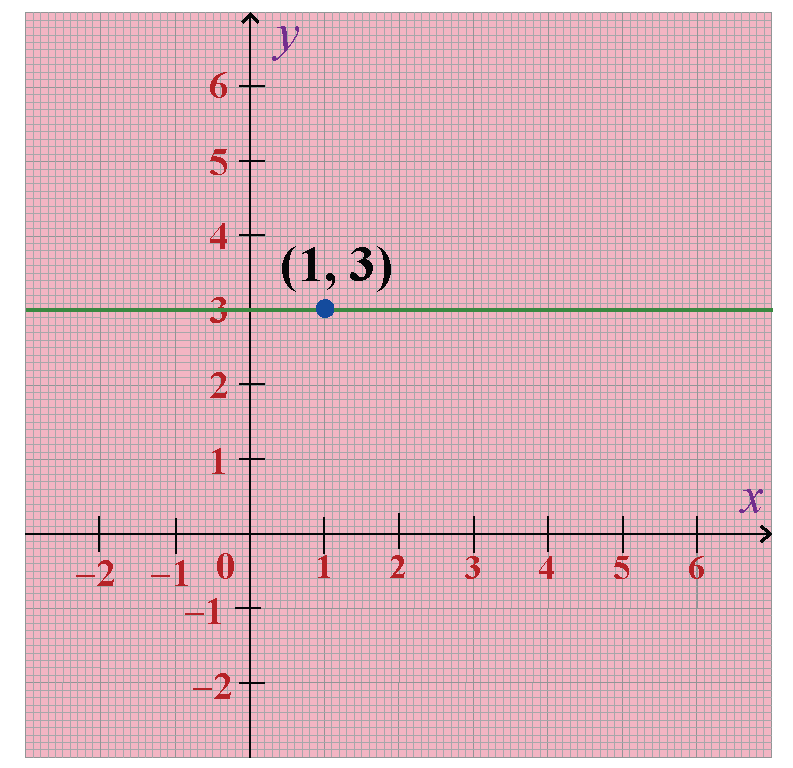Solution

The line parallel to $$x$$ axis has 0 slope.

So, $$m=0$$.

Let $$x_1=1$$ and $$y_1=3$$.

By point-slope form, the equation of the line is $${y-y_1=m(x-x_1)}$$

\begin{align}y-3&=0\times(x-1)\\y-3&=0\\y&=3\end{align}

 $$\therefore$$ The equation of the line is $$y=3$$.Think Tank
1. Which of the following graphs may represent the equation $${y+2= -2(x-1)}$$?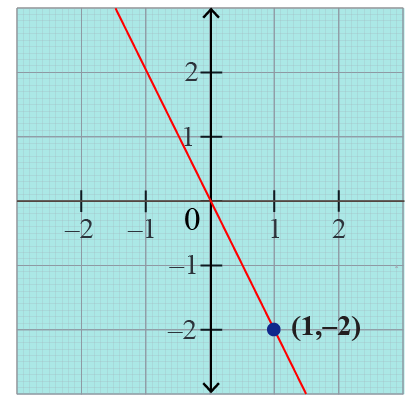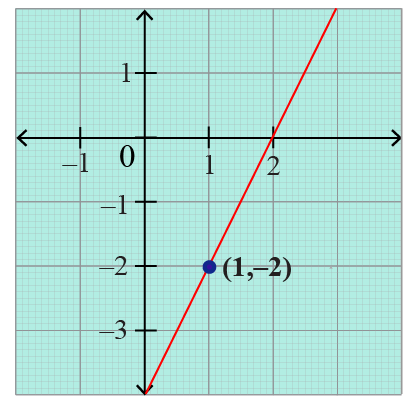## Interactive Questions

Here are a few activities for you to practice.

## Let's Summarize

We hope you enjoyed learning about the Equation of a Straight Line with the examples and practice questions. Now, you will be able to easily solve problems on the concentric circles.

At Cuemath, our team of math experts is dedicated to making learning fun for our favorite readers, the students!

Through an interactive and engaging learning-teaching-learning approach, the teachers explore all angles of a topic.

Be it worksheets, online classes, doubt sessions, or any other form of relation, it’s the logical thinking and smart learning approach that we, at Cuemath, believe in.

## FAQs on Equation of a Straight Line

### 1. What is a straight line in math?

A straight line is a figure formed when two points $$A (x_1 , y_1)$$ and $$B (x_2 , y_2)$$ are connected with minimum distance between them, and both the ends extended to infinity.

### 2. Who invented the equation of a straight line?

René Descartes invented the equation of a straight line.

### 3. What is the formula for the equation of a straight line?

Generally, we represent a straight line using the formula: $$ax + by = c$$, where $$x$$ and $$y$$ are variables.

### 4. What is the y-intercept formula?

If a line intersects the $$y$$-axis at the point $$(0,c)$$, then $$c$$ is the $$y$$-intercept.

### 5. How do you find the slope and y-intercept of an equation?

The slope $$m$$ of a line joining two points $$(x_1,y_1)$$ and $$(x_2,y_2)$$ is given by $$m=\dfrac{y_2-y_1}{x_2-x_1}$$.

The $$y$$ intercept of a straight line $$ax + by = c$$ is found by substituting the $$x$$ as 0.

### 6. How do you graph a slope-intercept equation?

The slope-intercept form is given by $$y=mx+c$$. It is plotted on graph by joining the two points satisfying the equation.

### 7. What is the y-intercept in y = -2x?

The $$y$$-intercept in $$y=-2x$$ is 0.

### 8. How do you write an equation for a vertical and horizontal line?

The equation of a horizontal line passing through $$(a,b)$$ is of the form $$y=b$$.

The equation of a vertical line passing through $$(a,b)$$ is of the form $$x=a$$.

### 9. How do you find the equation of a straight line when given two points?

The equation of a straight line joining two points $$(x_1,y_1)$$ and $$(x_2,y_2)$$ is given by $$(y-y_1)=\dfrac{y_2-y_1}{x_2-x_1}(x-x_1)$$.

### 10. How do you find the equation of a line from a pair of straight lines?

A pair of straight lines gives us two equations of straight lines.

More Important Topics
Numbers
Algebra
Geometry
Measurement
Money
Data
Trigonometry
Calculus